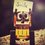# Need information

I posted this to some forum and got response , can somebody explain.

I think that when a charge system is put in a medium then the medium gets induced and exert force on the particle (test charge) thus net force is vector sum of forces by both medium and charge. this way force in particle due to charge should remain unaffected. correct me if I am wrong

The actual interaction between two charged particles through the exchange of photons which is a carrier of electromagnetic force. Your view is very very classical.Note by Kushal Patankar
6 years, 2 months ago

This discussion board is a place to discuss our Daily Challenges and the math and science related to those challenges. Explanations are more than just a solution — they should explain the steps and thinking strategies that you used to obtain the solution. Comments should further the discussion of math and science.

When posting on Brilliant:

• Use the emojis to react to an explanation, whether you're congratulating a job well done , or just really confused .
• Ask specific questions about the challenge or the steps in somebody's explanation. Well-posed questions can add a lot to the discussion, but posting "I don't understand!" doesn't help anyone.
• Try to contribute something new to the discussion, whether it is an extension, generalization or other idea related to the challenge.

MarkdownAppears as
*italics* or _italics_ italics
**bold** or __bold__ bold
- bulleted- list
• bulleted
• list
1. numbered2. list
1. numbered
2. list
Note: you must add a full line of space before and after lists for them to show up correctly
paragraph 1paragraph 2

paragraph 1

paragraph 2

[example link](https://brilliant.org)example link
> This is a quote
This is a quote
    # I indented these lines
# 4 spaces, and now they show
# up as a code block.

print "hello world"
# I indented these lines
# 4 spaces, and now they show
# up as a code block.

print "hello world"
MathAppears as
Remember to wrap math in $$ ... $$ or $ ... $ to ensure proper formatting.
2 \times 3 $2 \times 3$
2^{34} $2^{34}$
a_{i-1} $a_{i-1}$
\frac{2}{3} $\frac{2}{3}$
\sqrt{2} $\sqrt{2}$
\sum_{i=1}^3 $\sum_{i=1}^3$
\sin \theta $\sin \theta$
\boxed{123} $\boxed{123}$

Sort by:

I am confused with respect to this too. Consider this problem:

1. Two identical point charges are kept 1$m$ distance apart and the electrostatic force between them is measured as $F_1$. Now a thin(thickness$\approx 1mm$) plastic sheet is introduced in the space between the charges. The force is measured again and found to be $F_2$. Then:

A. $F_1>F_2$

B. $F_1

C. $F_1=F_2$

D. Depends on nature of plastic.

If I remember right, the answer is $A$... But I thought it was $C$, no idea how to explain it.

- 6 years, 2 months ago

The answer must be C the plastic between the doesn't affect the charges since it is neutral.

Have you attended the counseling of IISC Raghav

- 6 years, 2 months ago

no, if it was a dielectric effect will be there, (unless it was negligibly thin) (or sheet was infinitely large)

think about it , the dipoles will align themselves as per electric field which is non uniform in magnitude (let us assume it is made of dipoles)

then, at all intermediate points, the positive and negative ends of adjacent dipoles will cancel each other (overlap) (like two barmagnets joined together) whereas at the boundary on either side, there will be excesss charge (like the poles)

(however, in the capacitor dielectric we deal with , the thing is so large and hence electric field is nearly independent of distance for each charged side $\frac {\sigma}{\epsilon}$ _

- 6 years, 2 months ago

What if it was a dielectric?

- 6 years, 2 months ago

I think, it would affect the force between them if the sheet was a dielectric.

- 6 years, 2 months ago

Even if the sheet was dielectric then also it would have no effect. Actually the charges must be inside the dielectric for some effect to take place

- 6 years, 2 months ago

No, I did not go. They also allowed me to attend in July. But they haven't given any other information. Is that what they told you too?

- 6 years, 2 months ago

Yes, that's what they told me too. But now when we have to pay fees for IISC

- 6 years, 2 months ago

I don't know when we have to pay the fees. They haven't mentioned anything about it in the mail. My father sent them a mail regarding the fee payment, they have not replied yet.

- 6 years, 2 months ago